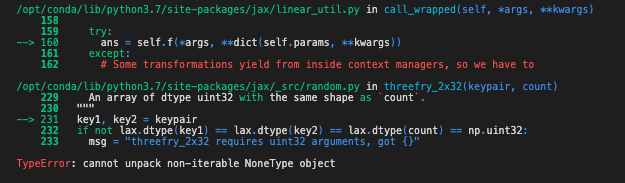# Substitute() breaks for lda model

I am running a plain vanilla latent dirichlet allocation model with Numpyro, and I am interested in investigating results once I fix the topic concentration (beta) parameters. I have noticed that 1) if I use substitute() or condition() trying to substitute in a site that does not actually exist in the model, the model runs from scratch and does not throw any error. This can be quite confusing, so I was wondering whether it is intentional; 2) if I substitute in a site that does exist, condition() works while substitute() breaks. As i want to treat beta parameters as data, I thought substitute() would be the most appropriate here, so I wonder what I am doing wrong.

Here the model:

``````def hmc_simple_model(W, K, alpha, eta):
D, V = jnp.shape(W)
N = W.sum(axis = 1)

with numpyro.plate("topics", K):
# topic-word distributions
beta = numpyro.sample("beta", dist.Dirichlet((eta) * jnp.ones([V])))

with numpyro.plate("docs", D):
# document-topic distributions
theta = numpyro.sample("theta", dist.Dirichlet(alpha*jnp.ones([K])))
ThetaBeta = jnp.matmul(theta, beta)

distMultinomial = dist.Multinomial(total_count = N, probs = ThetaBeta)
with numpyro.plate("hist", D):
numpyro.sample("obs", distMultinomial, obs = W)
``````

Minimal code for data simulation:

``````D = 200 # n. documents
V = 3000 # n. terms in the vocabulary
eta = 0.2 # hyperparameter for topic concentrations
alpha = 1 # hyperparameter for document distributions
K = 10 # number of topics

# topic-term distributions
beta = dist.Dirichlet(eta * jnp.ones([V])).expand([K]).sample(Key(8))

# document-topic distributions
theta = dist.Dirichlet(alpha * jnp.ones([K])).expand([D]).sample(Key(89))

thetabeta = jnp.matmul(theta,beta)
N = jnp.floor(dist.Uniform(100,200).expand([D]).sample(Key(2)))

# doc-term matrix
W = dist.Multinomial(total_count = N, probs = thetabeta).sample(Key(20))
``````

Minimal code for running the model:

``````n_warmup = 500
n_samples = 500

# substitute (or condition) with non-existent site: this runs
hmc_model_sub = condition(hmc_simple_model, {"not_a_site" : 5})
nuts_kernel_sub = NUTS(hmc_model_sub)
mcmc_sub = MCMC(nuts_kernel_sub, num_warmup=n_warmup,
num_samples=n_samples)
mcmc_sub.run(rng_key = Key(75), W = W, eta=eta, K=K, alpha=alpha)

# substitute with existent site: this does not run (while condition runs)
hmc_model_sub = substitute(hmc_simple_model, {"beta" : beta})
nuts_kernel_sub = NUTS(hmc_model_sub)
mcmc_sub = MCMC(nuts_kernel_sub, num_warmup=n_warmup,
num_samples=n_samples)
mcmc_sub.run(rng_key = Key(75), W = W, eta=eta, K=K, alpha=alpha)
``````

And below the error I get when trying substitute() with an existent site:1 Like

It is a bit tricky to explain why the error happens. For your usage case, I believe that `condition` is what you want (`substitute` plays no role in defining a model but it can be used for intervention - like what you did). I’ll add a fix to bypass the error (by telling init strategies skip those substituted sites - please help me open a github issue for thisbecause it would be nice to have a better error message) but I think that it is not what you want.

I guess you can also use block to block the `beta` site (this solution is faster than the `condition` solution)

``````hmc_model_sub = block(
substitute(hmc_simple_model, {"beta" : beta}), hide=["beta"])
``````
1 Like

The difference between `condition` and `substitute` is a little unclear in the documentation. And Pyro does not have a `substitute` poutine, I think, so it may be worth clarifying further.

Would it be also possible to write

``````hmc_model_sub = block(
condition(hmc_simple_model, {"beta" : beta}), hide=["beta"])
``````

and if so, should the performance be similar?

1 Like

Yes, the performance should be similar, and using `block(condition(...))` is better. `substitute` is mainly used by internal algorithms and we won’t recommend using it for modeling. Under the hood, `condition` makes sure that the conditioned sample site is observed, as mentioned in docs.

2 Likes

Just to clarify, the github issue would be about allowing `substitute` to work directly without the need for `block`, or about throwing a better error when it does not work directly?

I think the issue is to raise a better error/warning message when an init strategy is used with a `substitute`d model.